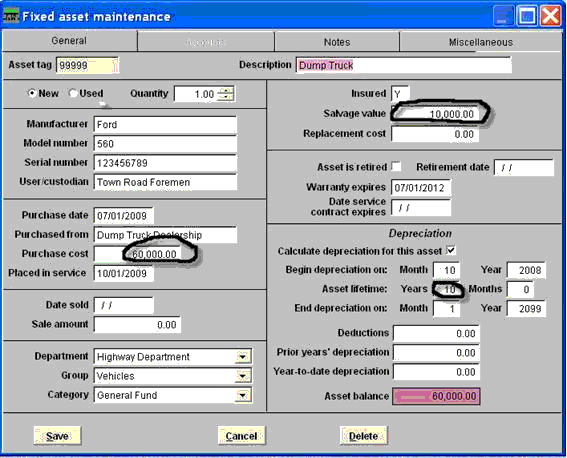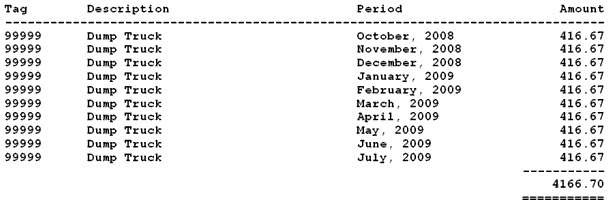# Fixed Assets Support Document

Open as: PDF or Word Document

## Straight Line Depreciation Method

NEMRC Fixed Asset uses the Straight Line Depreciation Method.

When calculating depreciation in the NEMRC Fixed Asset module, you can print a report with a monthly amount of depreciation, most of the time your assets will be depreciated with a total of 12 months worth of depreciation unless your asset was placed in service during the year you are running the calculation.

The depreciation amount is calculated by taking the purchase price of an asset subtracted by the salvage value divided by the asset lifetime then divided by 12 (for the number of months in a year).

Example: 60,000.00-10,000.00 = 50,000.00 / 10 years /12 = 416.67 per monthBecause begin depreciation on date is October of 2008, the calculation was for 10 months